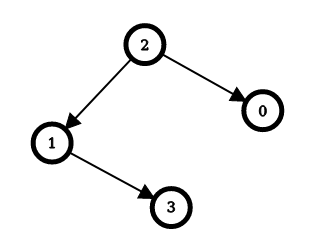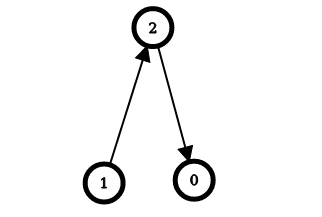# 战局详情

412 / 3028Juruoer120:55:210:10:130:30:210:45:21 2

# 题目及解答

8039. 使数组成为递增数组的最少右移次数

• 1 <= nums.length <= 100
• 1 <= nums.length <= 100
• nums 中的整数互不相同。

1. 整个数组本身递增，此时无需右移。
2. 整个数组可以分为前后两个递增数组，并且后一个递增数组的最后一个元素小于前一个递增数组的第一个元素，此时需要将后一个递增数组移动到前一个递增数组前面，即移动 后一个递增数组的长度 次。

2856. 删除数对后的最小数组长度

nums 中下标在 ij 处的元素删除。剩余元素按照原来的顺序组成新的数组，下标也重新从 0 开始编号。

• 1 <= nums.length <= 1e5
• 1 <= nums[i] <= 1e9
• nums非递减 数组。

1. 存在一个个数最多的数字，出现了$k$ 次，并且$k \ge \lfloor \frac{n}{2} \rfloor$，那么上述代码中每一次的 top1都是该数剩余的数量，也就是说这个数可以和其他所有数配对删除，并且有可能有剩余。此时的结果为$n - (n - k) \times 2$

2. 存在一个个数最多的数字，出现了$k$ 次，并且$k < \lfloor \frac{n}{2} \rfloor$，那么可以被一直配对，直至只剩一个数或全被配对完，此时结果为$n \mod 2$

6988. 统计距离为 k 的点对

• 2 <= coordinates.length <= 50000
• 0 <= xi, yi <= 1e6
• 0 <= k <= 100

• (0, 1)：(1 XOR 4) + (2 XOR 2) = 5 。
• (2, 3)：(1 XOR 5) + (3 XOR 2) = 5 。

100041. 可以到达每一个节点的最少边反转次数

• 2 <= n <= 1e5
• edges.length == n - 1
• edges[i].length == 2
• 0 <= ui == edges[i] < n
• 0 <= vi == edges[i] < n
• ui != vi
• 输入保证如果边是双向边，可以得到一棵树。1. 它到它的子节点要么无须反转（父节点指向子节点），要么需要反转 1 次（子节点指向父节点）
2. 它到它的父节点要么无须反转（子节点指向父节点），要么需要反转 1 次（父节点指向子节点）